# Quantification of network structural dissimilarities

## Abstract

Identifying and quantifying dissimilarities among graphs is a fundamental and challenging problem of practical importance in many fields of science. Current methods of network comparison are limited to extract only partial information or are computationally very demanding. Here we propose an efficient and precise measure for network comparison, which is based on quantifying differences among distance probability distributions extracted from the networks. Extensive experiments on synthetic and real-world networks show that this measure returns non-zero values only when the graphs are non-isomorphic. Most importantly, the measure proposed here can identify and quantify structural topological differences that have a practical impact on the information flow through the network, such as the presence or absence of critical links that connect or disconnect connected components.

## Introduction

Quantifying dissimilarities and determining isomorphisms among graphs are fundamental open problems in computer science, with a very long history1,2,3,4,5,6,7,8,9,10,11,12,13,14,15. The graph isomorphism problem consists in deciding whether two graphs are identical, presenting a one-to-one correspondence between its components. This problem holds a special place in the complexity theory field, as no polynomial time algorithm is still known. Thus, its complexity remains undefined since the mid-70s. A recent work proposed a quasi-polynomial time algorithm16, which checks subsections of the graphs for isomorphism, through a series of simple means. However, the problem remains, for highly symmetric structures that are still very expensive to compute17,18.

In practice, the quantification of graph dissimilarities brings much more information about the graphs than the binary answer to the graph isomorphism problem. Similarity measures have many uses due to the current widespread use of networks in social sciences, medicine, biology, physics and so on19,20,21,22,23,24,25,26,27,28,29,30. They can help, among many other examples, to discriminate between neurological disorders by quantifying functional and topological similarities31, to find structurally more similar molecules that are more likely to exhibit similar properties, for drug design32, and to quantify changes in temporal evolving networks22.

Most methods for graph comparison have shown to be efficient for specific purposes, but the information they provide is often limited or incomplete. Important structural differences are missed or underestimated, because the measure employed considers graph properties that only partially describe the graphs33.

Regarding network functionality, it is important that a dissimilarity measure captures and adequately quantifies topological differences. A good dissimilarity measure should have the ability to recognize the different roles of links and nodes, considering disconnections and other structural conditions.

The goal of this work is to propose a discriminative and computationally efficient metric to distinguish and quantify graph dissimilarities. We define a dissimilarity metric able to identify and quantify topological differences. The main idea to measure the dissimilarity, D(G, G′), of two graphs containing directed or undirected links is to associate to each structure a set of probability distribution functions (PDFs), representing all node’s connectivity distances, and compare them, by standard information-theoric metrics. We consider three distance-based PDF vectors in a three-term function. The first term compares networks, through their network’s distance distributions, capturing global topological differences. The second term compares the connectivity of each node and how each element is connected throughout the network, by looking at the node’s distances distributions. The last term analyses the differences in the way this connectivity occurs, through the analysis of the alpha centrality.

The D-measure (D) allows one to compare networks efficiently and with high precision. We prove that isomorphic graphs present a zero distance. Extensive computational experiments show that, D, do not present any counterexample when recognizing non-isomorphic structures. We also find that the measure is able to characterize the evolution of dynamical systems, being able to identify the small-world region in the Watts–Strogatz process (WS) and phase transitions in Erdös–Rényi (ER) network’s evolution. Considering real networks, D evaluates the goodness of the adjustment of network models and predicts their critical percolation probabilities.

## Results

### D-measure

We introduce D with a simple example. Figure 1 displays three networks with nine nodes and nine links, representing different topologies: N1 has no disconnections, N2 has one disconnected node and N3 is disconnected into three connected components. Table 1 depicts results for two popular distance measures, Hamming (H)34 and graph edit distance (GED)35. As it can be seen in this example, they do not capture relevant topological differences, returning the same distance value for all comparisons and missing the fact that N3 is totally disconnected.

A good measure should return a higher distance value between N1 and N3, than between N1 and N2. Differently of N2, that has only one disconnected node, N3 presents three connected components, completely interrupting the information flow through the network. Interesting comparisons are also pairs N1–N3 and N2–N3. The measure should recognize N3 as more similar to N2 than to N1, as both N3 and N2 have disconnected elements.

We begin by defining the concept of network node dispersion (NND). The NND is a measure of the heterogeneity of a graph G in terms of connectivity distances. We qualify a network as heterogeneous when it possesses a high diversity of node-distance patterns and, consequently, a high NND value. NND will be used in the definition of D(G, G′). It is computed by the Jensen–Shannon divergence, a dissimilarity measure among N PDFs36.

To perform a highly precise comparison, instead of using vectors in which the elements are numbers (for example, the number of links of each node), we consider vectors in which the elements are PDFs; specifically, the distance distribution in each node i, Pi={pi(j)}, with pi(j) being the fraction of nodes that are connected to node i at distance j. The set of N node-distance distributions, {P1PN}, contains detailed information of the topology of the network, in a compact way. From this set, the network’s degree distribution, the network’s distance distribution and several other features can be deduced (see Supplementary Note 1).

Considering a network with N nodes, the set of N distance distributions {P1PN}, is normalized by log(d+1), being d the network’s diameter. Then, NND is defined as: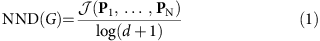with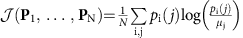and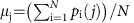being the Jensen–Shannon divergence and the average of the N distributions, respectively.

We illustrate the properties of NND with two numerical experiments, using well-known network models.

The first one considers 100 ER networks37 generated by randomly connecting pairs of nodes with probability P. Different network sizes (N=102, 103 and 104) and different probability values are considered. At low P values the network consists of a set of small connected components and when increasing P above a critical value, Pc=1/N, the network collapses in a single large connected component, corresponding to the percolation transition. Figure 2a depicts how the NND detects this transition for all sizes considered, being Pc the last point before the peak. We also note that the maximum NND value (P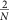) possesses a very low variation as N increases (Supplementary Fig. 1).

The second experiment consists of 100 realizations of the WS rewiring model38. The number of nodes (N=103) and number of links are constant, corresponding to an average degree equal to 10. Figure 2b shows NND versus the rewiring probability, P, in logarithmic scale. We observe that the NND allows delimiting the small-world region between its maximum and minimum values: maximum NND indicates maximum connectivity heterogeneity, whereas minimum NND indicates that the nodes are more homogeneously connected.

As shown by the previous examples, NND captures relevant features of a network and thus it can be used for network comparison. However, most k-regular networks (graphs in which all nodes have degree k) possess NND=0. To define a general dissimilarity measure, it is important to properly discriminate them.

To take this into account, we also consider for the definition of the dissimilarity measure, the difference between the graphs averaged node-distance distributions (network’s distance distribution), μG and μG′, and the comparison between the α-centrality values of the graphs and their complements39, computed through the Jensen–Shannon divergence (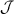) (see Supplementary Note 2).

Then, the dissimilarity measure proposed is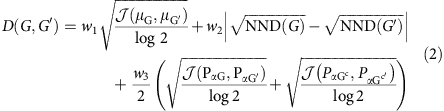where N and M are the sizes of G and G′, respectively, and Gc indicates the complement of G. As the NND is always <1 and(PG, PG′)/log 2≤1 then, 0≤D(G, G′)<1. w1, w2 and w3 are arbitrary weights of the terms where w1+w2+w3=1; however, after extensive experimentation we selected the following weights w1=w2=0.45 and w3=0.1 as the most appropriate to quantify structural dissimilarities in networks. Supplementary Note 3 shows that the choice of the weights does not change the metric character and presents a discussion regarding the weights selection. This approach can be easily adapted to compare networks of different number of nodes, as discussed in Supplementary Note 4.

Defined in this way, D captures global and local graphs dissimilarities. The first term compares averaged connectivity node’s patterns, corresponding to the so-called graph distance distribution28. Graphs sharing the same distance distribution present the same diameter, average path length (APL) and other connectivity features.

The second term analyses the heterogeneity of the nodes. Graphs presenting the same NND are graphs that have the same connectivity distance profile.

The third term considers the centrality of each node, taken into account each node’s direct and indirect connectivity span. When considering the graph’s complement, the measure also captures the effect of disconnected nodes. This term is the only one able to discriminate between complete graphs of different sizes and also among other distance-regular structures such as the Desargues and dodecahedral graphs (see Supplementary Fig. 2a).

D(G, G′) identifies and properly quantifies structural topological differences, which affect the information flow through the networks. This can be seen in Fig. 1 and Table 1, in which increasing topological differences correspond to higher D-values.

### Isomorphism

By performing extensive experiments in synthetic and real-world networks, we show that D(G, G′) recognizes isomorphic graphs, returning non-zero values when the graphs are non-isomorphic.

We note that D(G, G′)=0 only if G and G′ have the same graphs distance distribution, the same NND and the same α-centrality vector. However, there is no guarantee that D returns a non-zero value for all non-isomorphic networks. In other words, it is possible to obtain D(G, G′)=0 even if G and G′ are not isomorphic. To investigate this limitation, we analysed all non-isomorphic graphs of size 6, 7, 8 and 9. For graphs with 20 nodes, we focused on the worst cases for D, k-regular connected graphs with degrees varying from 2 to 11. Finally, we also generate all non-isomorphic trees with 20 and 21 nodes. After 1012 comparisons, results demonstrate the high accuracy of the proposed measure for recognizing the non-isomorphic condition, without any counter-example (see Data availability in Methods for instances and algorithms).

Most importantly, we observe that, from a computational perspective, the time complexity of the algorithm is polynomial, as it relies on the computation of all shortest paths length, that is known to be a polynomial problem40, that by using Fibonacci heaps can be implemented in O(E+N logN)41. The Hamming distance is computed in polynomial time, only when nodes are labelled, as it consists in a matrix difference O(N2). However, the problem with H is the lack of information, as it only considers the number of missing links and not their role in the topology structure. In the case of GED, its computation corresponds to a NP-Hard problem2, being very unlikely to expect a polynomial approach to compute it. Besides the major drawback of an exponential computational time, the usefulness of its results as a measure of dissimilarity is at least questionable. As it can be seen in Fig. 1 and Table 1, neither H or GED can properly detect and manage network disconnections. Supplementary Note 5 and Supplementary Tables 1 and 2 present algorithms found in the literature, either to solve the isomorphism problem or to compute a dissimilarity measure between networks; this compilation briefly describes their main characteristics, drawbacks and results.

### Classical models

We consider five networks with 20 nodes and 40 links: a four-regular network (R), a random network (ER), two small-world structures with P-values corresponding to the lowest and highest NND (WSMIN and WSMAX), and a scale-free Barabási–Albert (BA) network with parameter m=2 (ref. 42).

The lowest D-value is obtained between ER and WSMIN. This is expected due to the fact that the WS process transforms a k-regular lattice into a random structure by rewiring links. D recognizes the small difference between them, as the intrinsic memory of the WS process does not allow the network to evolve to a pure ER structure43. However, when these two structures are compared against other networks, the differences captured by D show no statistical significance. See Supplementary Table 3 for values and confidence intervals.

In contrast, the highest D-value is obtained for BA and WSMAX, followed by BA and R. The BA network corresponds to the most complex structure from the five here studied. In terms of node distances distributions, the BA structure possesses low node-distance heterogeneity, as a great number of nodes are connected to hubs, in a similar way. Thus, D considers BA closer to R than WSMAX. WSMAX corresponds to a stage in the WS process in which the number of shortcuts created in the network generates a decrease in the APL, increasing the node-distance heterogeneity. Besides the low values of APL, BA structures are known to present low clustering coefficient, features also present in ER and WSMIN. D acknowledges this fact by locating them closer to BA. Figure 3 depicts a schematic representation of the networks obtained through a multidimensional scaling map of the D-values between all pairs of networks presented by increasing averaged values over 1,000 experiments.

For the following example, we first consider synthetic networks generated by WS and ER processes. Figure 4a depicts the dissimilarity value for all pairs of networks of size N=103 constructed during the WS process. The first row and column represent the distance between all graphs and the initial lattice. The maximum dissimilarity value, not considering comparisons with the initial lattice, coincide with the maximum and minimum NND values, delimiting the small-world region. It can be seen that networks corresponding approximately to P<10−3 are very similar between each other and they become gradually more dissimilar to networks generated with higher P-values. For networks in the region 2 × 10−1<P<1, they are similar to each other, but very dissimilar to initial networks. Finally, networks corresponding to probabilities in the interval 10−3<P<2 × 10−1 are dissimilar to networks of both extremes of the process, delimiting the small-world region.

Figure 4b shows the dissimilarity values D for all pairs of ER networks of size N=103. D clearly captures the topological phase transition at Pc. As expected, higher values are obtained when comparing networks with P below and above the critical value. We also note that networks with P<Pc are more similar among each other than networks with P>Pc.

### Percolation on real networks

The phase transition captured by the dissimilarity function in the ER model represents the bond percolation threshold on complete graphs; however, as this measure captures abrupt changes in distances within the network, it also captures the existence of a percolation threshold in real networks. Figure 5 shows how D captures the largest percolation transition in the Power Grid network (Pc≈0.6632) and also a double phase transition characterized by two small peaks in the susceptibility function at P≈8 × 10−5 and P≈6 × 10−3, as depicted in the two small figures.

We propose here an algorithm based on the hypothesis that, when looking for the phase transition, two networks in the subcritical or supercritical phases present smaller D-values than a pair of graphs with one in each phase. By applying a bisection method-like procedure, we obtain good approximations of the percolation transition with a low number of simulations. We compare our results against the Monte Carlo (MC) algorithm proposed by Newman and Ziff44. We follow the instructions used by Radicchi45, where an extensive empirical experiment was performed using MC.

The algorithm begins with two probabilities, β and α, respectively, on the supercritical and subcritical phases. We compute the mean value of these probabilities Pm=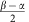and through a series of simulations we estimate the distance between their correspondent averaged graph structures. If D(Gm, Gα)>D(Gm, Gβ) then β=Pm else α=Pm, when the distance between β and α reaches a precision value (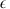), the algorithm stops returning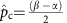. Table 2 depicts results for a set of real networks. Supplementary Note 6 presents a pseudo-code and a detailed explanation of the experiment.

In terms of computational complexity, after the first iteration, our algorithm computes s different networks per iteration and each corresponding NND. Thus, per iteration, our algorithm has a complexity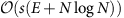, considering=0.01, α=0 and β=1, we need to perform seven iterations. For the specific example of the Power Grid network, with s=100, our algorithm needs 5,500 s, against the 35,000 s of the MC with 10,000 iterations (CPU times of both algorithms can be improved with a good Pc approximation value). By increasing s and reducing, we can improve the algorithm precision, which can also be used as a warm start for the MC procedure.

### Model selection

We consider here the problem of choosing the most appropriated model to simulate real systems. In this experiment, we use D to compare real networks with well-known null models including, Molloy–Reed (MR)46, Maslov–Sneppen (MS)47 and dk model25. MR is a null model that preserves the degree distribution of the network, but the connection structure is lost. MS is a null model where links are randomly rewired. Its default setting considers 4|E| rewiring procedures. However, exists an appropriate number of rewiring operations from which MS can be considered equivalent to MR. Finally, we consider the dk models for different k-values (1.0, 2.0, 2.1 and 2.5). k=1.0 generates networks preserving the degree sequence and, as it can be seen in Supplementary Note 7, it is equivalent to MR and MS null models. k=2.0 preserves the degree sequence and degree correlation; k=2.1 also preserves the clustering coefficient and finally k=2.5 includes the clustering spectrum.

Each model is run 30 independent times and the averaged D-values are presented in Fig. 6. When preserving only the degree sequence, the null models capture some topological features; however, they have no information regarding node’s correlation and global connectivity patterns. It can be seen from Fig. 6 that, as expected, D decreases as parameter k increases.

It is worth noting that, in most cases, transitions from k=1.0 to k=2.0 and from k=2.0 to k=2.1 present significative differences (see confidence intervals in Supplementary Table 4). That is not always the case for transitions between k=2.1 to k=2.5. Results for the Petster (C) network show that models considering k=2.1 are closer to the real network than models k=2.5; this can be the case of an outlier network as discussed in ref. 25. After the analysis of the generated networks, we could verify that k=2.1 produce networks with closer APL (3.558) than their k=2.5 counterpart (3.502) and both overestimate the network diameter 16.21 and 15.6; these are average results over 30 runs. The original network has APL=3.588 and diameter 10.

It is interesting noticing that the Power Grid and Euroroad networks show significative higher distances to the dk model when compared with all other real networks. This poor adjustment of the dk model to the Power Grid network is also discussed in refs 25, 48.

### Distances between real networks

We use the dissimilarity measure D to compare real-world networks. We consider 16 data sets of 9 network types: computer, online contact, communication, human contact, infrastructure, lexical, metabolic, social and co-authorship. All networks are freely available at The Koblenz Network Collection49 (see description in Supplementary Note 8).

Figure 7a depicts D-values between all pairs of networks. Remarkably, Social Networks appear to be very similar to each other, in good agreement with previous observations50. In addition, we can observe that CAIDA, a computer type network, is similar to communication, social, co-authorship and the human contact infections socio-patterns network. The infrastructure networks (Power Grid and Euroroad) are the most different with respect to the entire group, but similar to each other. Both networks present particular characteristics, as scarcity due to physical constraints, presenting neither a scale-free nor a classical small-world behaviour51,52. A tree-like structure, which is also possible to visualize in Fig. 7a, is a common feature in these networks. D captures this structural pattern differentiating them from all other topologies.

We compare these networks (Power Grid and Euroroad) with other well-known tree-like structures, as are the case of networks constructed via the horizontal visibility graph53, from fractional Brownian motion (fBm) time series, with different Hurst exponents (H)54. We found that these networks posses significantly lower distances to fBm networks than to the dk model. This can be seen in Fig. 7b, in which we compare distances between the Power Grid network with networks generated by dk model and also with an fBm (H=0.14) network (see Supplementary Note 9).

### Brain networks

As a final application, we perform a study to compare brain networks constructed through electroencephalography exams (EEG). The data contain measurements from 64 electrodes placed on the subject’s scalps sampled at 256 Hz (3.9 ms epoch) during 1 s55. The full data set contains 120 trials for 122 subjects; however, as some samples are incomplete, we consider only the 107 subjects with complete trials (39 control and 68 alcoholic samples).

For each subject, a weighted network of the entire brain is created following the method described in ref. 56. However, instead of using a linear correlation measure between the time series, we transform them into a graph via horizontal visibility graph algorithm53 and we consider the correlation between each pair of regions as given by 1 minus the dissimilarity D (1−D(G, G′)). The resulting network represents the weighted similarity between brain regions, allowing comparisons between individual brain networks.

By using this straightforward methodology, we are able to detect two regions of the brain called ‘nd’ and ‘y’, where the weight of the connections between these regions is higher in control than in alcoholic networks, as shown in Fig. 8. Supplementary Fig. 9 depicts the results of applying the same methodology but considering the Hamming distance, in which it is possible to see that it is not capable of distinguishing between the groups.

## Discussion

D is a highly precise network dissimilarity measure, based on three distance-based PDF vectors extracted from the graphs and defined as a three-term function. It compares, through the Jensen–Shannon divergence, topological differences between networks. Through extensive numerical experiments, we show that D appropriately captures topological differences between networks and returns D=0, when comparing isomorphic graphs. Non-zero D-values indicate a non-isomorphic condition and represent a quantification of the topological difference between them.

D is able to identify the small-world region in a WS process and phase transitions in ER network’s evolution. Considering real systems, D evaluates the goodness of the adjustment of network models and predicts their critical percolation probabilities.

One aspect we must point out is that the use of D to compare sparse graphs, as it is the case of real-world networks, implies in processing dense graphs when computing the α-centrality of their graph’s complements, increasing the computational cost. However, as the use of the third term (α-centrality) is only strictly necessary to distinguish highly regular structures, D can be computed avoiding the third term of the equation, without significant precision loss.

D also have many practical uses, that among many others, we can mention applications in image and pattern recognition and in the characterization of time-evolving networks. D can be employed in the design of accurate classifiers for biological networks and is a promising tool to study different aspects of multilayer networks.

### Data availability

All relevant data and algorithms are publicly available at https://github.com/tischieber/Quantifying-Network-Structural-Dissimilarities.

How to cite this article: Schieber, T. A. et al. Quantification of network structural dissimilarities. Nat. Commun. 8, 13928 doi: 10.1038/ncomms13928 (2017).

Publisher’s note: Springer Nature remains neutral with regard to jurisdictional claims in published maps and institutional affiliations.

## References

1. 1

Kelmans, A. K. Comparison of graphs by their number of spanning trees. Discrete Math. 16, 241–261 (1976).

2. 2

Garey, M. R. & Johnson, D. S. Computers and Intractability: a Guide to the Theory of NP-Completeness W. H. Freeman & Co. (1979).

3. 3

IEEE, T., Pattern Anal Bunke, H. & Shearer, K. A graph distance metric based on the maximal common subgraph. Pattern. Recogn. Lett. 19, 255–259 (1998).

4. 4

Fernandez, M. L. & Valiente, G. A graph distance metric combining maximum common subgraph and minimum common supergraph. Pattern. Recogn. Lett. 22, 753–758 (2001).

5. 5

Luo, B. & Hancock, E. R. Structural graph matching using the EM algorithm and singular value decomposition. IEEE T. Pattern. Anal. 23, 1120–1136 (2001).

6. 6

Raymond, J. W., Gardiner, E. J. & Willett, P. Heuristics for similarity searching of chemical graphs using a maximum common edge subgraph algorithm. J. Chem. Inf. Comp. Sci. 42, 305–316 (2002).

7. 7

Conte, D. et al. Thirty years of graph matching in pattern recognition. Int. J. Pattern Recogn. 18, 265–298 (2004).

8. 8

Dehmer, M. et al. A similarity measure for graphs with low computational complexity. Appl. Math. Comput. 182, 447–459 (2006).

9. 9

Przulj, N. Biological network comparison using graphlet degree distribution. Bioinformatics 23, E177–E182 (2007).

10. 10

Zager, L. A. & Verghese, G. C. Graph similarity scoring and matching. Appl. Math. Lett. 21, 86–94 (2008).

11. 11

Gao, X., Xiao, B., Tao, D. & Li, X. A survey of graph edit distance. Pattern Anal. Appl. 13, 113–129 (2010).

12. 12

Soundarajan, S., Eliassi-Rad, T. & Gallagher, B. in Proceedings of the 2014 SIAM International Conference on Data Mining, 1037–1045 (2014).

13. 13

Fischer, A. et al. Approximation of graph edit distance based on Hausdorff matching. Pattern Recogn. 48, 331–343 (2015).

14. 14

Aliakbary, S. et al. Distance metric learning for complex networks: towards size-independent comparison of network structures. Chaos 25, 023111 (2015).

15. 15

Bougleux, S. et al. A quadratic assignment formulation of the graph edit distance. Preprint at https://arxiv.org/abs/1512.07494v1 (2015).

16. 16

Babai, L. Graph isomorphism in quasipolynomial time. Preprint at https://arxiv.org/abs/1512.03547v2 (2016).

17. 17

Savage, N. Graph matching in theory and practice. Commun. ACM 59, 12–14 (2016).

18. 18

Borgwardt, K. M. Graph Kernels (PhD Thesis, Fakultät für Mathematik, Informatik und Statistikder LudwigMaximiliansUniversität (2007).

19. 19

Boccaletti, S. et al. Complex networks: structure and dynamics. Phys. Rep. 424, 175–308 (2006).

20. 20

Arenas, A. et al. Synchronization in complex networks. Phys. Rep. 469, 93–153 (2008).

21. 21

Barabasi, A. L., Gulbahce, N. & Loscalzo, J. Network medicine: a network-based approach to human disease. Nat. Rev. Genet. 12, 56–68 (2011).

22. 22

Carpi, L. et al. Structural evolution of the Tropical Pacific climate network. Eur. Phys. J. B 85, 1434–6028 (2012).

23. 23

Schieber, T. A. & Ravetti, M. G. Simulating the dynamics of scale-free networks via optimization. PLoS ONE 8, e80783 (2013).

24. 24

Taylor, D. et al. Topological data analysis of contagion maps for examining spreading processes on networks. Nat. Commun. 6, 7723 (2015).

25. 25

Orsini, C. et al. Quantifying randomness in real networks. Nat. Commun. 6, 8627 (2015).

26. 26

De Domenico, M., Nicosia, V., Arenas, A. & Latora, V. Structural reducibility of multilayer networks. Nat. Commun. 6, 6864 (2015).

27. 27

Menche, J. et al. Uncovering disease-disease relationships through the incomplete interactome. Science 347, 1257601 (2015).

28. 28

Schieber, T. A. et al. Information theory perspective on network robustness. Phys. Lett. A 380, 359–364 (2016).

29. 29

Verma, T., Russmann, F., Araújo, N. A. M., Nagler, J. & Herrmann, H. J. Emergence of coreperipheries in networks. Nat. Commun. 7, 10441 (2016).

30. 30

Çolak, S., Lima, A. & González, M. C. Understanding congested travel in urban areas. Nat. Commun. 7, 10793 (2016).

31. 31

Calderone, A. et al. Comparing Alzheimers and Parkinsons diseases networks using graph communities structure. BMC Syst. Biol. 10, 1–10 (2016).

32. 32

Morrow, J. K., Tian, L. & Zhang, S. Molecular Networks in Drug Discovery. Crit. Rev. Biomed. Eng. 38, 143–156 (2010).

33. 33

Costa, L. et al. Characterization of complex networks: a survey of measurements. Adv. Phys. 56, 167–242 (2007).

34. 34

Hamming, R. W. Binary codes capable of correcting deletions, insertions, and reversals. AT&T Tech. J. 10, 147–160 (1950).

35. 35

Sanfeliu, A. & Fu, K. S. A distance measure between attributed relational graphs for pattern recognition. IEEE T. Syst. Man Cyb. 13, 353–363 (1983).

36. 36

Lin, J. Divergence measures based on the Shannon entropy. IEEE T. Inform. Theory 37, 145–151 (1991).

37. 37

Erdös, P. & Rényi, A. On random graphs. Publ. Math. 6, 290–297 (1959).

38. 38

Watts, D. J. & Strogatz, S. H. Collective dynamics of small-world networks. Nature 393, 440–442 (1998).

39. 39

Bonacich, P. Power and centrality: a family of measures. Am. J. Sociol. 92, 1170–1182 (1987).

40. 40

Dijkstra, E. W. A note on two problems in connexion with graphs. Numer. Math. 1, 269–271 (1959).

41. 41

Fredman, M. L. & Tarjan, R. E. Fibonacci Heaps and Their Uses in Improved Network Optimization Algorithms. J. ACM 34, 596–615 (1987).

42. 42

Albert, R. & Barabási, A. Statistical mechanics of complex networks. Rev. Mod. Phys. 74, 47–97 (2002).

43. 43

Carpi, L. et al. Analyzing complex networks evolution through Information theory quantifiers. Phys. Lett. A 375, 801–804 (2011).

44. 44

Newman, M. E. J. & Ziff, R. M. Efficient Monte Carlo algorithm and high-precision results for percolation. Phys. Rev. Lett. 85, 4101 (2000).

45. 45

Radicchi, F. Predicting percolation thresholds in networks. Phys. Rev. E 91, 010801 (2015).

46. 46

Molloy, M. & Reed, B. The size of the giant component of a random graph with a given degree sequence. Comb. Probab. Comput. 7, 295–305 (1998).

47. 47

Maslov, S. & Sneppen, K. Specificity and stability in topology of protein networks. Science 296, 910–913 (2002).

48. 48

Jamakovic, A. et al. How small are building blocks of complex networks. Preprint at https://arxiv.org/abs/0908.1143v2 (2015).

49. 49

Kunegis, J. KONECT—The Koblenz Network Collection. In Proc. Int. Conf. on World Wide Web Companion, 1343–1350 (2013).

50. 50

Newman, M. E. J. & Park, J. Why social networks are different from other types of networks. Phys. Rev. E 68, 036122 (2003).

51. 51

Subelj, L. & Bajec, M. Robust network community detection using balanced propagation. Eur. Phys. J. B 81, 353–362 (2011).

52. 52

Watts., D. J. Small Worlds: The Dynamics of Networks between Order and Randomness Princeton Univ. Press (2003).

53. 53

Luque, B., Lacasa, L., Ballesteros, F. & Luque, J. Horizontal visibility graphs: exact results for random time series. Phys. Rev. E 80, 046103 (2009).

54. 54

Gonçalves, B. A., Carpi, L., Rosso, O. A. & Ravetti, M. G. Time series characterization via horizontal visibility graph and information theory. Phys. A 464, 93–102 (2016).

55. 55

Begleiter, H. EEG Database Data Set https://archive.ics.uci.edu/ml/datasets/EEG+Database (1995).

56. 56

Joudaki, A., Salehi, N., Jalili, M. & Knyazeva, M. G. EEG-based functional brain networks: does the network size matter? PLoS ONE 7, e35673 (2012).

## Acknowledgements

We wish to acknowledge the referees for the constructive comments and Pol Colomer-de-Simón. Research partially supported by FAPEMIG, CNPq (Brazil). C.M. acknowledges partial support from Spanish MINECO (FIS2015-66503-C3-2-P) and ICREA ACADEMIA. A.D.-G. acknowledges financial support from MINECO, Projects FIS2012-38266 and FIS2015-71582, and from Generalitat de Catalunya Project 2014SGR-608. P.M.P. acknowledges support from the ‘Paul and Heidi Brown Preeminent Professorship in ISE, University of Florida’ and RSF grant 14-41-00039.

## Author information

Authors

### Contributions

T.A.S., L.C. and M.G.R. conceived the experiments. T.A.S. and M.G.R. conducted the experiments. All authors analysed the results, wrote and reviewed the manuscript.

### Corresponding author

Correspondence to Martín G. Ravetti.

## Ethics declarations

### Competing interests

The authors declare no competing financial interests.

## Supplementary information

### Supplementary Information

Supplementary Figures, Supplementary Notes, Supplementary Tables and Supplementary References. (PDF 9840 kb)

## Rights and permissions

Reprints and Permissions

• ### Model simplification for supervised classification of metabolic networks

• Ilaria Granata
• , Mario R. Guarracino
• , Valery A. Kalyagin
• , Ichcha Manipur
•  & Panos M. Pardalos

Annals of Mathematics and Artificial Intelligence (2020)

• ### Framework based on communicability to measure the similarity of nodes in complex networks

• Dan Chen
• , Housheng Su
•  & Gui-Jun Pan

Information Sciences (2020)

• ### Brain network similarity: methods and applications

• , Fabrice Wendling
•  & Mahmoud Hassan

Network Neuroscience (2020)

• ### Examining the variability in network populations and its role in generative models

• Viplove Arora
• , Dali Guo
• , Katherine D. Dunbar
•  & Mario Ventresca

Network Science (2020)

• ### Enhancing transport properties in interconnected systems without altering their structure

• Arsham Ghavasieh
•  & Manlio De Domenico

Physical Review Research (2020)